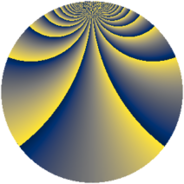# Properties

 Label 345.2.wLevel $345$ Weight $2$ Character orbit 345.w Rep. character $\chi_{345}(7,\cdot)$ Character field $\Q(\zeta_{44})$ Dimension $480$ Newform subspaces $1$ Sturm bound $96$ Trace bound $0$

# Related objects

## Defining parameters

 Level: $$N$$ $$=$$ $$345 = 3 \cdot 5 \cdot 23$$ Weight: $$k$$ $$=$$ $$2$$ Character orbit: $$[\chi]$$ $$=$$ 345.w (of order $$44$$ and degree $$20$$) Character conductor: $$\operatorname{cond}(\chi)$$ $$=$$ $$115$$ Character field: $$\Q(\zeta_{44})$$ Newform subspaces: $$1$$ Sturm bound: $$96$$ Trace bound: $$0$$

## Dimensions

The following table gives the dimensions of various subspaces of $$M_{2}(345, [\chi])$$.

Total New Old
Modular forms 1040 480 560
Cusp forms 880 480 400
Eisenstein series 160 0 160

## Trace form

 $$480q + 8q^{6} + O(q^{10})$$ $$480q + 8q^{6} + 8q^{13} + 72q^{16} - 96q^{23} - 24q^{25} - 16q^{26} - 176q^{28} + 8q^{31} + 40q^{32} - 8q^{35} - 56q^{36} - 88q^{37} - 40q^{41} - 220q^{42} + 56q^{46} - 152q^{47} + 32q^{48} + 128q^{50} - 220q^{52} - 48q^{55} - 88q^{57} + 32q^{58} - 88q^{61} - 96q^{62} - 112q^{70} + 24q^{71} - 40q^{73} + 32q^{75} - 176q^{76} - 40q^{77} + 16q^{78} - 396q^{80} + 48q^{81} + 88q^{82} - 400q^{85} - 8q^{87} - 104q^{92} + 64q^{93} - 244q^{95} - 48q^{96} + 200q^{98} + O(q^{100})$$

## Decomposition of $$S_{2}^{\mathrm{new}}(345, [\chi])$$ into newform subspaces

Label Dim. $$A$$ Field CM Traces $q$-expansion
$$a_2$$ $$a_3$$ $$a_5$$ $$a_7$$
345.2.w.a $$480$$ $$2.755$$ None $$0$$ $$0$$ $$0$$ $$0$$

## Decomposition of $$S_{2}^{\mathrm{old}}(345, [\chi])$$ into lower level spaces

$$S_{2}^{\mathrm{old}}(345, [\chi]) \cong$$ $$S_{2}^{\mathrm{new}}(115, [\chi])$$$$^{\oplus 2}$$